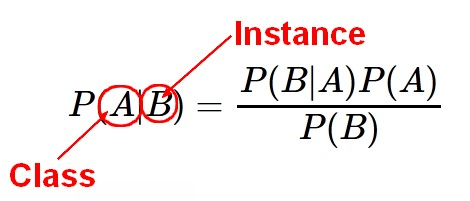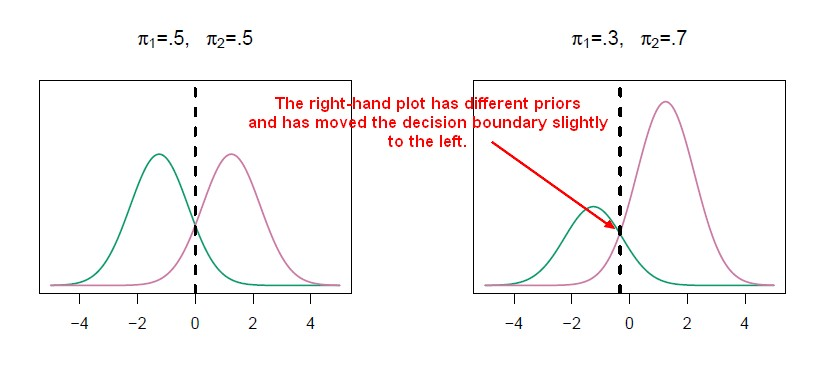# Statistics - Quadratic discriminant analysis (QDA)

When the variances of all X are different in each class, the magic of cancellation doesn't occur because when the variances are different in each class, the quadratic terms don't cancel.

And therefore , the discriminant functions are going to be quadratic functions of X.

Quadratic discriminant analysis uses a different covariance matrix for each class.

Quadratic discriminant analysis is attractive if the number of variables is small.

## Discriminant Function

$$\delta_k(x) = - \frac{1}{2} (x - \mu_k)^T \sum^{-1}_k ( x - \mu_k) + log(\pi_k)$$

As there's no cancellation of variances, the discriminant functions now have this distance term that involves $\sum_k$ , which is for the kth class.

There's :

• a term to do with the prior probability,
• a determinant term that comes from the covariance matrix.

It gives a curved discriminant boundary.

Recommended PagesData Mining - Naive Bayes (NB)

Naive Bayes (NB) is a simple supervised function and is special form of discriminant analysis. It's a generative model and therefore returns probabilities. It's the opposite classification strategy...Statistics Learning - Discriminant analysis

Discriminant analysis is a classification method. In discriminant analysis, the idea is to: model the distribution of X in each of the classes separately. use what's known as Bayes theorem to flip...